﻿ Digital Circuits and Systems - Circuits i Sistemes Digitals (CSD) - EETAC - UPCBachelor's Degree in Telecommunications Systems and in Network EngineeringType 74HCT283

1. Specifications

Design a 4-bit adder (adder_4bit) using carry-lookahead (CLA) technique in a hierarchical structure of multiple VHDL files. In Fig. 1 there is the circuit's symbol and truth table. The algorithm is: S = A + B + Cin. The commercial 4-bit adder standard chip 74HCT283 is similar.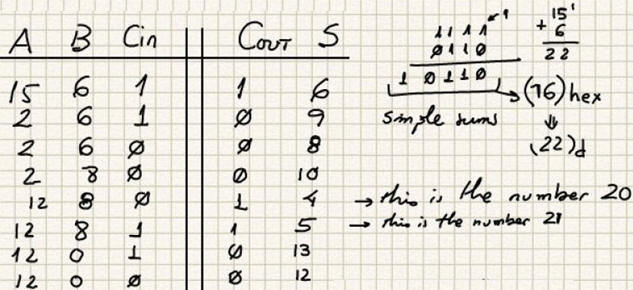Fig. 1. Adder_4bit symbol and truth table.  f for the 4-bit adder and its truth table.

Fig. 2 shows an example of timing diagram.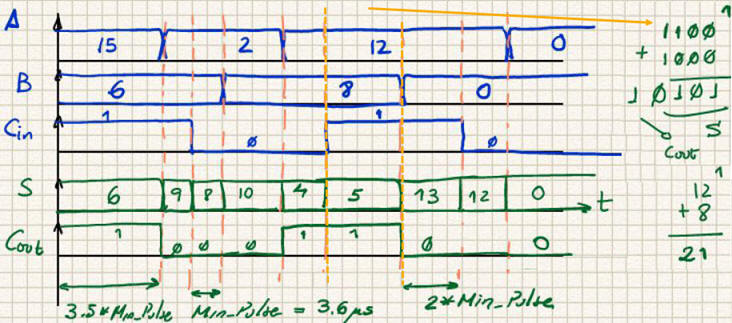Fig. 2. This is an example of a timing diagram representing some operations.

The idea of the carry-lookahead to reduce the propagation time in addition operations is explained in Wikipedia and also in many books. For instance: Ercegovac, M., Lang, T., Moreno, J. H., "Introduction to Digital Systems", John Wiley & Sons, 1999). It includes slides: Chapter 10 is on arithmetic circuits.

2. Planning

Structural and hierarchical plan C2 based on the carry-lookahead technique. Fig. 3 shows the concept to reduce the circuit's propagation time to only 6·tPg. instead of 12·tPg. as is was the case in the ripple-carry Adder_4bit.Fig. 4. The initial idea:an extra circuit Carry_generator to obtain all the four carrys required by the Adder_1bit chips in a single step (3 levels of gates).

We observe in fig. 4 that the Adder_1bit circuit do not need to be a full 1-bit adder because the logic for Co is not used but replaced by the new circuit Carry_generator. Thus, it can be simplified.

And, at the same time, we can focus our attention on how the equation Co = f(ai, bi, ci) is written in algebra.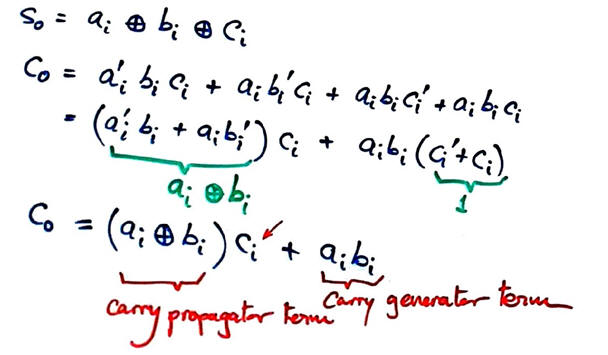Fig. 5. The idea of carry propagator (pi) and carry generator (gi) in the 1-bit adder unit.

Thus, Carry_generator output equations for the four Adder_1bit units can be deduced as shown in Fig. 6. All of them consist of only two levels of gates. In this circuit NOT literals are not required.Fig. 6. Carry_generator equations.

To be able to chain Adder_4bit when constructing larger adder strcutures, we must pay attention to the final Cout, it can also be explained in terms of group or module propagator (P) and generator (G).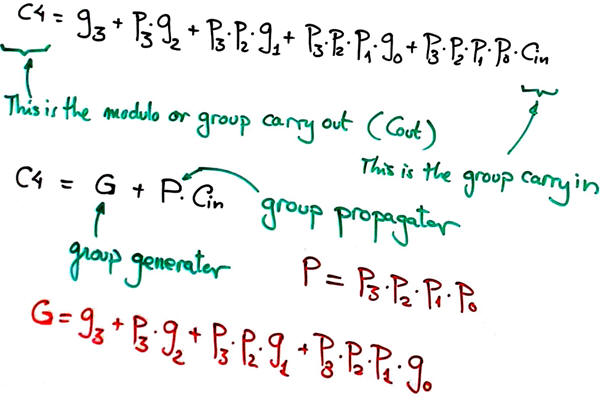Fig. 7. Outputs group propagator (P) and group generator (G) will be included in the symbol so that this Adder_4bit will be chainable along with other levels of carry generator components. For instance, read in Wikipedia how an Adder_16bit works.

In this way, the circuit idea in Fig. 3 can be optimised in Fig. 8, containing only the component Carry_generator and logic gates. From the equations we observe that the circuit's propagation time is P = 4·tPg.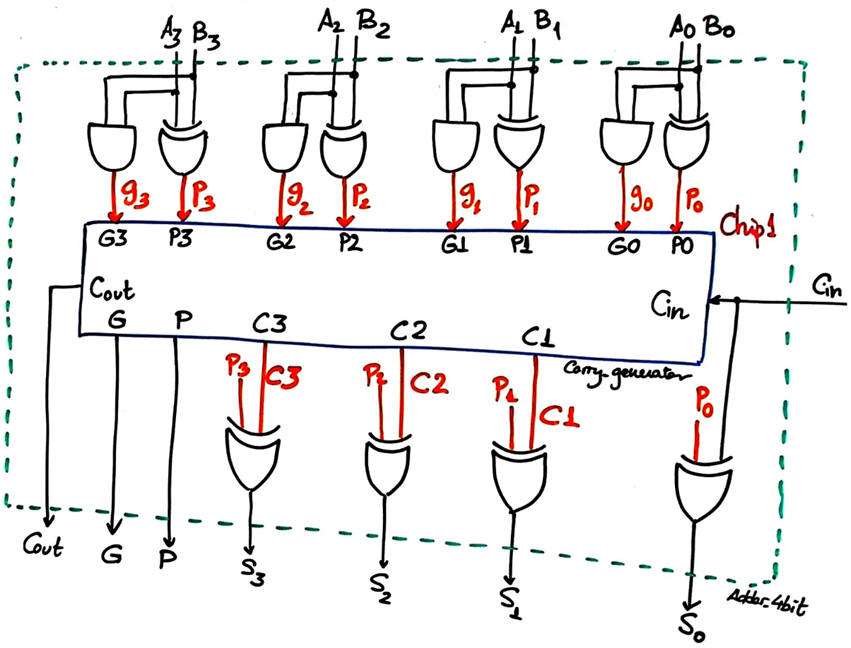Fig. 8. Optimised internal architecture for the CLA Adder_4bit. Two VHDL files will be required.

Project location:

3. Developing the project using EDA tools

From the planning section we can translate Fig. 8 into a VHDL file Adder_4bit.vhd, along with the component Carry_generator.vhd.

We can pick up an Intel PLD, for instance the MAXII EPM2210F324C3 and develop a multiple file project as shown in the Fig. 9 RTL schematic.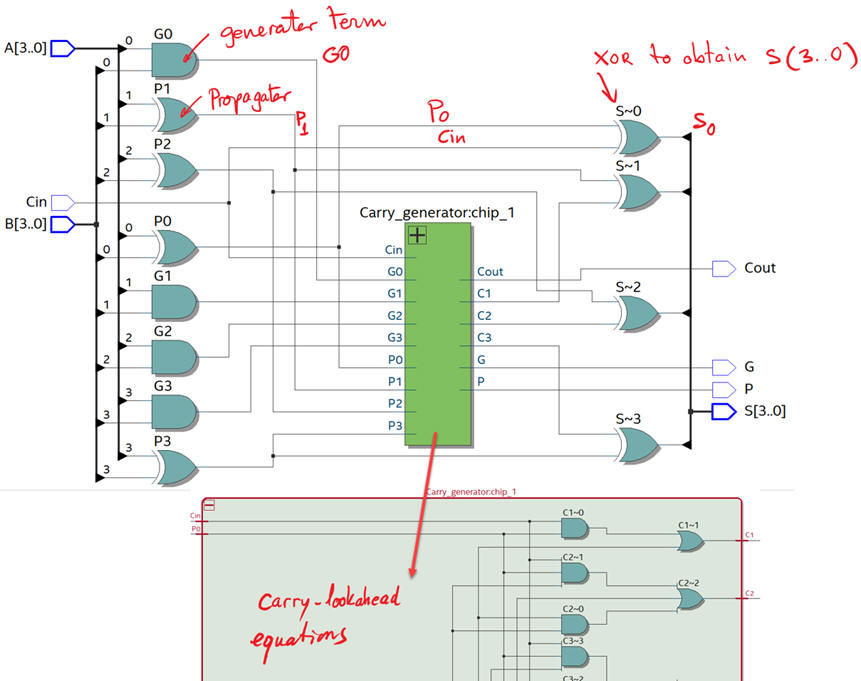Fig.9. RTL view of the hierarchical structure once synthesised using EDA tools. It looks like as expected from Fig. 8. It is somewhat similar to the classic 74HCT283.

In MAX II CPLD devices, logic gates and circuits are syntheised using logic elements.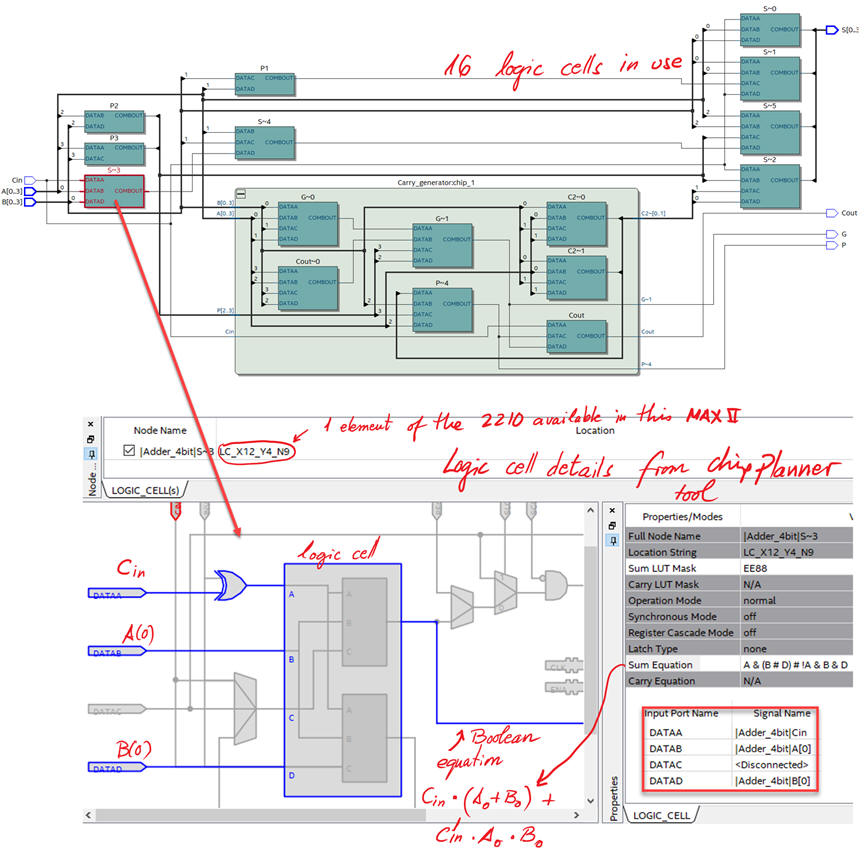Fig.10. Tecnology view when using a MAXII CPLD . How many logic elements are used? This view and the project summary tell us that 16 logic elements are used.

4. Testing and validating the design

The testbench fixture allows us to perform VHDL simulations for testing the unit-under-test.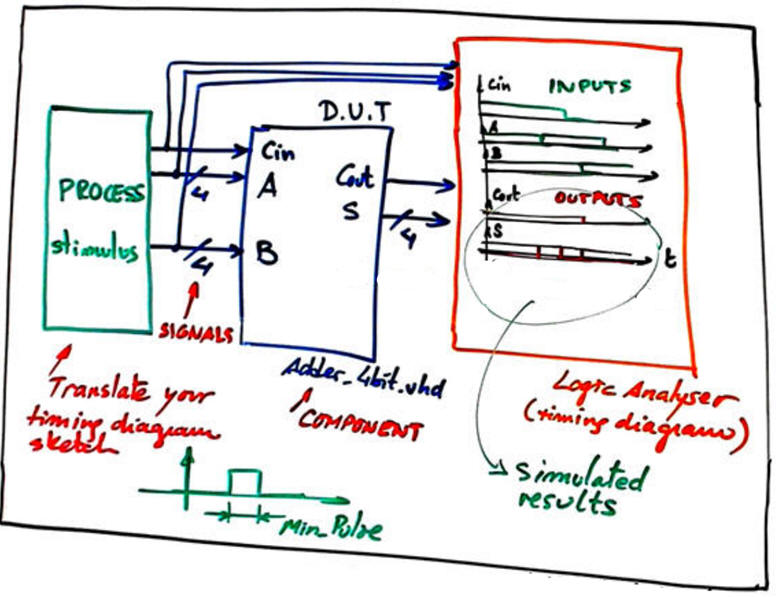Fig.11. VHDL testbench fixture.

Translating the testbench into VHDL Adder_4bit_tb.vhd, we can run the VHDL simulation EDA tool to obtain and discuss the timing diagram. This is an example of resulting waveforms.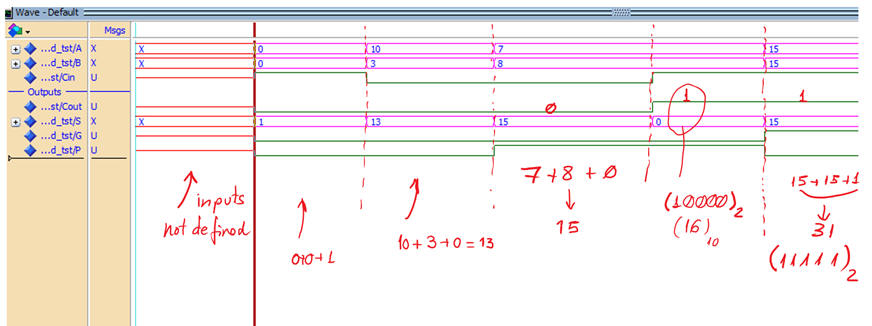Fig. 12. Example of a test results.

5. Testing (gate-level)

The same VHDL fixture applies for this architecture because the unit under test is the same.Fig.13. Gate-level measurements in a given signal transition for measuring propagation times.

Timing analyser spreadsheet will show us the worst-case scenario, the longest delay, and thus, the maximum speed of the circuit.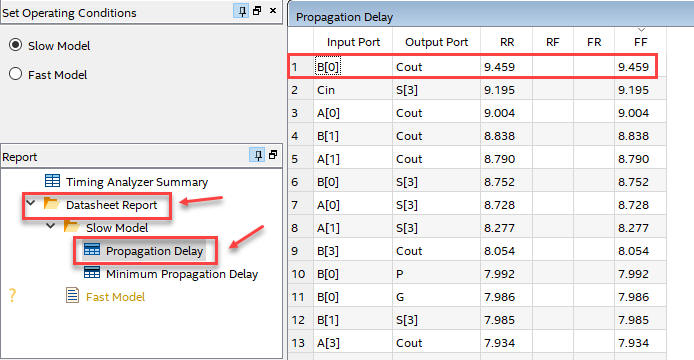Fig.14. Timing analyser results. In this example the longuest signal propagation path is from B(0) to Cout, representing tP = 9.46 ns. From this measurement we can deduce what is the maximum frequency of operation fMAX (representing the minimum value for Min_Pulse, or the maximum ratio at which inputs can be switched) for a given target chip. In this case fMAX = 1/2·tP = 52.8 MHz. Thus it is capable of performing 52.8 Mops (millions of operations per second).

It is time to compare both implementations of the same circuit (1) ripple-carry Adder_4bit and (2) CLA Adder_4bit studied here. Which one is faster? Which one uses more resources?

For instance, in a project of 16-bit adder is discussed comparing performances when designed using a ripple-carry and carry-lookahead architectures.

6. Report

6. Prototyping

Use training boards and perform laboratory measurements to verify how the circuit works.

Other materials of interest

(optional) Plan B.- Behavioural. It is too complex and difficult to explain and systematise for the learning goals of this introductory CSD course). This is the same circuit where the truth table itself (behavioural description) has to be written in VHDL using this sketch. (A single VHDL file project). The VHDL file will be named the same Adder_4bit.vhd. This is the file Adder_4bit.vhd. This is another shorter version of the same file, just to show you the flexibility of the description language. This is the RTL of the flat -behavioural- architecture.

Represented in Fig. 15 is the RTL of the flat (behavioural, algorithmic) architecture.Fig. 15. RTL schematic from a behavioural (algorithmic, truth table) point of view.